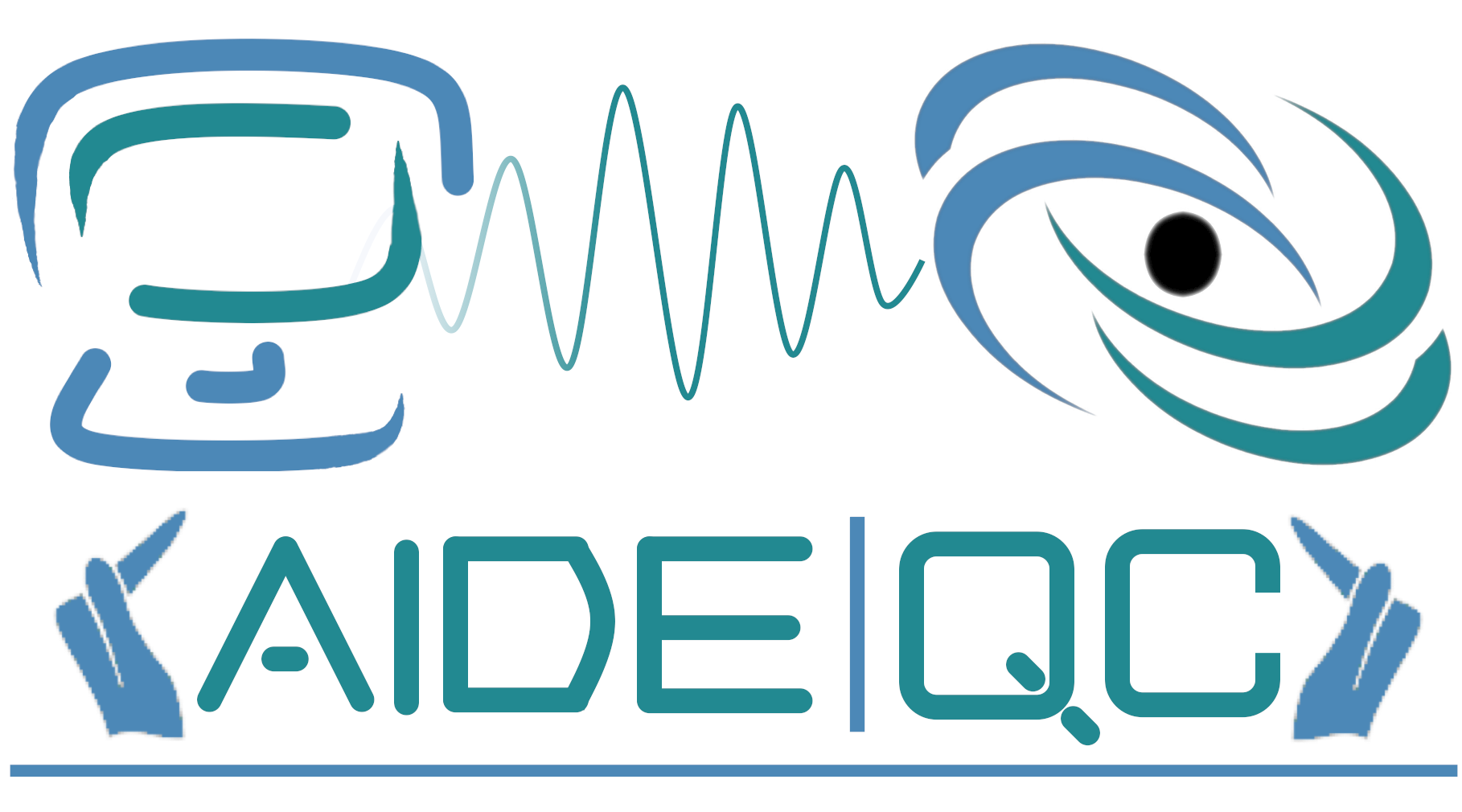#AIDE-QC

Advanced Integrated Development Environment for Quantum Computing

# Data Model

## Variational Data Model ¶

Due to the ubiquity of variational quantum algorithms in near-term quantum computation, the QCOR language specification puts forward novel data-types that enable efficient programming of quantum-classical variational algorithms. We specify the structure of these types here:

### HeterogeneousMap ¶

HeterogeneousMap is used across the QCOR variational API as a container for a heterogeneous mapping of data. Specifically, this data type should map string keys to values of any type. This can be accomplished in C++ for instance via a std::map<std::string, std::any>.

### Operator ¶

Operator is an interface that encapsulates the behavior of a quantum mechanical operator. It exposes an API for algebraic operations on multiple Operators, as well as a method for observing an input unmeasured quantum kernel (e.g. state-preparation ansatz for variational algorithms). Operators can be constructed from a string representation or a HeterogeneousMap of options. Observation via an exposed observe() method takes as input a quantum kernel and returns a list or vector of measured quantum kernels, whereby measurements for return kernel i are dictated by the Operator’s ith term (e.g. X0X1 would append Hadamard gates followed by measure instructions). The Operator is ultimately just a high-level interface and is meant to be subclassed by concrete operator types. The default types that must be provided by language implementors are PauliOperator and FermionOperator, any further sub-type definitions are up to the implementation. The base-level Operator subtype is the PauliOperator since it is required ultimately for any calls to observe(). In addition to the Operator, the language specification also mandates and OperatorTransform interface, which is meant to be sub-typed by concrete realizations that can transform higher-level Operators to the PauliOperator. Moreover, this interface can be used for any general transformation on Operators (e.g. symmetry reductions, etc.).

The language extension should provide utility functions for generating PauliOperator and FermionOperator instances efficiently. The implementation should provide X(int), Y(int), and Z(int) function calls to generate the x,y,z pauli matrices, respectively. The implementation should provide adag(int) and a(int) to provide creation and annhilation FermionOperator instances.

The language extension should provide the following Operator creation API:

• createOperator(expression : string) : Operator, which defaults to PauliOperator creation and requires a string representation of the desired PauliOperator
• createOperator(type: string, expression : string) : Operator, which creates an Operator of the provided type (pauli or fermion) and requires a string representation of the desired Operator
• createOperator(type: string, options : HeterogeneousMap) : Operator, which creates an Operator of the provided type (pauli or fermion) from a provided set of options represented as a HeterogeneousMap (e.g. create a chemistry Hamiltonian from the basis set and geometry).

### ObjectiveFunction ¶

ObjectiveFunction is a representation of a parameterized function y = f(U(x'),x,O) where x denotes a list of scalar parameters, O denotes an Operator, and U(x') denotes a quantum kernel whose behavior is controlled by x' (related to x as defined by user). ObjectiveFunction is initialized with Operator and quantum kernel. As a default the ObjectiveFunction evaluates the expectation value of O with respect to U at a given x' (e.g. <0|U(x')^\dagger \hat{O} U(x')|0>). ObjectiveFunction can be extended to provide specialized processing of the expectation values for non-VQE use cases. Using the default and extended ObjectiveFunction, in tandem with the Optimizer, one can succinctly implement variational quantum algorithms, for example VQE.

### Optimizer ¶

Optimizer represents a data-type encapsulating an optimization strategy executed on a given ObjectiveFunction. Specically the Optimizer exposes a member function, optimize, that takes as input an ObjectiveFunction argument and computes its optimal value and parameters which are returned as either a pair or tuple. The language extension should also enable the ability to use the optimize() method on any general function instance, as long as the function argument structure is correct. The correct structure should be double(std::vector<double>,std::vector<double>&) (as an example in C++), where the first vector is the input parameters and the second is a reference to the gradient vector.

### Example Usage ¶

__qpu__ void ansatz(qreg q, double theta) {
X(q);
auto exponent_op = X(0) * Y(1) - Y(0) * X(1);
exp_i_theta(q, theta, exponent_op);
}

int main(int argc, char **argv) {
// Allocate 2 qubits
auto q = qalloc(2);

// Create the Deuteron Hamiltonian
auto H = 5.907 - 2.1433 * X(0) * X(1) - 2.1433 * Y(0) * Y(1) + .21829 * Z(0) -
6.125 * Z(1);

// Create the objective function
auto objective = createObjectiveFunction(ansatz, H, q, 1);

// Create a qcor Optimizer
auto optimizer = createOptimizer("nlopt");

// Optimize the above function
auto [optval, opt_params] = optimizer->optimize(objective);

// Print the result
printf("energy = %f\n", optval);

return 0;
}